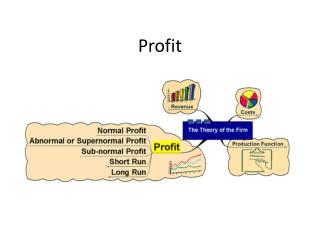Download PresentationProfit

# Profit

Download Presentation## Profit

- - - - - - - - - - - - - - - - - - - - - - - - - - - E N D - - - - - - - - - - - - - - - - - - - - - - - - - - -
##### Presentation Transcript

1. Profit

2. Learning Targets: • Distinguish between economic and normal profit. • Explain why a firm will continue even when it earns zero economic profit? • Why is economic profit is also called supernormal profit or abnormal profit. • Explain positive and negative profit.

3. Profit • Profit = TR – TC • The reward for enterprise • Profits help in the process of directing resources to alternative uses in free markets

4. Profit • Economic Profit= Total revenue- economic cost(implicit cost + explicit cost) • Normal Profit- the minimum amount required to keep a firm running • Revenue= economic cost or economic Profit = zero • This is also known as break-even point of a firm. • allocation

5. Why a firm continues to operate even when earning zero economic profit? • Note That: • When a firm is earning normal profit, it has covered all its opportunity cost (implicit cost) and will continue to operate

6. Positive and Negative Profit: • Economic profit can be zero, positive of negative • supernormal or abnormal profit: • Positive economic profit is also referred to as supernormal or abnormal profit. • This is because it involves profit over and above the economic profit.

7. Profit To summarize: • Positive economic profit = TR>economic cost, the firm supernormal profit • Zero economic profit= TR=economic cost, the firm earns normal profit • Negative economic profit = TR<economic profit, the firm makes a loss (sub-normal profit)

8. Goals of firms

9. Learning Targets • Explain the goal of profit maximization where the difference between TR and TC is minimized or when MC=MR

10. Profit Maximization • Involves determining the levels of output that the firm should produce to make profit as large as possible. • Yet firms do not always make profit as revenue is not sufficient to cover all costs

11. Profit maximization based on TR & TC Approach • This is based on the simple principle of TR-TC=economic profit • If the difference between TR and TCis positive, the firm is making abnormal profit • If the difference between TR and TC is = to 0, the firm is making normal profit • If the difference between TR and TC is negative, the firm is making a loss

12. Profit Max. using TR and TC with NO price control Normal econ profit TC-TR=0 Loss minimization TR<TC Profit maximization TR>TC

13. Profit Max using TR and TC- has price control Profit maximization -At point Qmax, profit is maximized -At point Q1 and Q2, ECON PROFIT=0 (break-even point) Loss Minimization -Firm is making a loss as TC>TR, However, loss is minimized at point Q1min

14. Profit Maximization Based of MC & MR • A firms profit max. rule is to choose to produce when MC=MR Why is this so? -Consider a firm is producing at point Q1 in both graphs, where MR>MC, if this firm increases its output by 1 unit, the MR>MC until it intercepts MR=MC. -but at Q2, MC>MR, therefore the firm must cut down its Q output

15. Explain the relationship between the given curves.